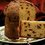# Inequalities

If $a$ and $b$ are positive numbers satisfying $a+b=1$, prove that $\left( a + \dfrac1a \right)^2 + \left( b + \dfrac 1b\right)^2 \geq \dfrac{25}2$.Note by Subham Subian
4 years, 9 months ago

This discussion board is a place to discuss our Daily Challenges and the math and science related to those challenges. Explanations are more than just a solution — they should explain the steps and thinking strategies that you used to obtain the solution. Comments should further the discussion of math and science.

When posting on Brilliant:

• Use the emojis to react to an explanation, whether you're congratulating a job well done , or just really confused .
• Ask specific questions about the challenge or the steps in somebody's explanation. Well-posed questions can add a lot to the discussion, but posting "I don't understand!" doesn't help anyone.
• Try to contribute something new to the discussion, whether it is an extension, generalization or other idea related to the challenge.

MarkdownAppears as
*italics* or _italics_ italics
**bold** or __bold__ bold
- bulleted- list
• bulleted
• list
1. numbered2. list
1. numbered
2. list
Note: you must add a full line of space before and after lists for them to show up correctly
paragraph 1paragraph 2

paragraph 1

paragraph 2

[example link](https://brilliant.org)example link
> This is a quote
This is a quote
    # I indented these lines
# 4 spaces, and now they show
# up as a code block.

print "hello world"
# I indented these lines
# 4 spaces, and now they show
# up as a code block.

print "hello world"
MathAppears as
Remember to wrap math in $$ ... $$ or $ ... $ to ensure proper formatting.
2 \times 3 $2 \times 3$
2^{34} $2^{34}$
a_{i-1} $a_{i-1}$
\frac{2}{3} $\frac{2}{3}$
\sqrt{2} $\sqrt{2}$
\sum_{i=1}^3 $\sum_{i=1}^3$
\sin \theta $\sin \theta$
\boxed{123} $\boxed{123}$

Sort by:

My latex is not working properly so I will just give a brief of the solution.

Apply AM-GM to get maximum value of ab. Apply QM-AM and substitute maximum value of ab to get minimum value of expression as ab is in denominator.

- 4 years, 9 months ago

can u use cauchy-schwarz inequality because this question was after this theorem

- 4 years, 9 months ago

plss

- 4 years, 9 months ago

QM-AM is a type of Cauchy-schwarz inequality.

- 4 years, 9 months ago

Another approach would be to note that the function $f(x)=\left(x+\frac 1x\right)^2$ is convex for all $x\in\Bbb R$, so using Jensen's inequality on $f$ with $a,b$, we have,

$\sum_{x\in\{a,b\}}\left(x+\frac 1x\right)^2=f(a)+f(b)\geq 2f\left(\frac{a+b}2\right)=2f(1/2)=2\left(\frac 12+2\right)^2=2\times\frac{25}4=\frac{25}2$

- 4 years, 9 months ago

Hint: This is equivalent to proving that $(a^2 + b^2) + \left( \dfrac1a + \dfrac1b \right) \geq 8.5$.

Now, for positive $a$ and $b$, what is the relationship between $a^2 + b^2$ and $a+b$? Similarly, what is the relationship between $\dfrac1a + \dfrac1b$ and $a+b$?

Read up Power mean inequality (qagh).

- 4 years, 9 months ago

thanks for yout help but i think it should (a^2+b^2)+(1/a^2+1/b^2)

- 4 years, 9 months ago

cause min. value of a^2+b^2 IS 1/2 and we need max.value of ab which is1/4 but after computing we get >=4.5 but if u take (a^2+b^2)+(1/a^2+1/b^2) we get the right answer ANYWAY THANKS FOR YOUR HELP!!!!!!!!!! THANKS A LOT!!!

- 4 years, 9 months ago

THANKS A LOT!!!

- 4 years, 9 months ago